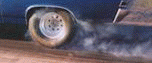Engineering ToolBox - Resources, Tools and Basic Information for Engineering and Design of Technical Applications!

# Car - Traction Force

## Adhesion and tractive force between car wheel and surface.The tractive force between a car wheel and the surface can be expressed as

F = μt W

= μt m ag   (1)

where

F = traction effort or force acting on the wheel from the surface (N, lbf)

μt = traction - or friction - coefficient between the wheel and the surface

W = weight or vertical force between wheel and surface (N, lbf))

m = mass on the wheel (kg, slugs)

agacceleration of gravity (9.81 m/s2, 32.17405 ft/s2)

### Traction Coefficients for normal Car Tires

Car Tires - Traction Coefficients
SurfaceTraction Coefficient
- μt -
Wet Ice 0.1
Dry Ice/Snow 0.2
Loose Sand 0.3 - 0.4
Dry Clay 0.5 - 0.6
Wet rolled Gravel 0.3 - 0.5
Dry rolled Gravel 0.6 - 0.7
Wet Asphalt 0.6
Wet Concrete 0.6
Dry Asphalt 0.9
Dry Concrete 0.9

### Example - Traction Force on an Accelerating Car

The maximum traction force available from one of the two rear wheels on a rear wheel driven car - with mass 2000 kg equally distributed on all four wheels - on wet asphalt with adhesion coefficient 0.5 - can be calculated as

Fone_wheel = 0.5 ((2000 kg)  (9.81 m/s2) / 4)

= 2453 N

The traction force from both rear wheels

Fboth_wheels = 2 (2452 N)

= 4905 N

Note! - that during acceleration the force from the engine creates a moment that tries to rotate the vehicle around the driven wheels. For a rear drive car this is beneficial by increased vertical force and increased traction on the driven wheels. For a front wheel driven car the traction force will be reduced during acceleration.

The maximum acceleration of the car under these conditions can be calculated with Newton's Second Law as

acar = F / m

= (4904 N) / (2000 kg)

= 2.45 m/s2

= (2.45 m/s2) / (9.81 m/s2)

= 0.25 g

where

acar = acceleration of car (m/s2)

The minimum time to accelerate from 0 km/h to 100 km/h can be calculated as

dt = dv / acar

= ((100 km/h) - (0 km/h)) (1000 m/km) (1/3600 h/s) / (2.4 m/s2)

= 11.3 s

where

dt = time used (s)

dv = change in velocity (m/s)

### Accelerating Car Calculator

This calculator can be used to calculate the maximum acceleration and minimum accelation time for a car on different surfaces.

## Related Topics

• ### Dynamics

Motion - velocity and acceleration, forces and torque.

## Related Documents

• ### Acceleration

Change in velocity vs. time used.
• ### Car - Required Power and Torque

Power, torque, efficiency and wheel force acting on a car.
• ### Car Acceleration

Car acceleration calculator.
• ### Car Fuel Consumption - liter/100 km

Calculate fuel consumption in liter per km - consumption chart and calculator.
• ### Cars - New vs. Old Car Cost Calculator

Calculate and compare the costs between owning a new vs. an old car.
• ### Driving Distances between European Cities

Driving distance between some major European cities.
• ### Electrical Vehicle Charging - Power vs. Voltage and Amps

EV Charging - AC vs. DC, single phase vs. three phase and power vs. voltage and amps.
• ### Friction - Friction Coefficients and Calculator

Friction theory with calculator and friction coefficients for combinations of materials like ice, aluminum, steel, graphite and many more.
• ### Fuel Consumption - mpg

Calculate fuel consumption in miles per gallon - mpg - calculator and consumption charts.
• ### Piston Engines - Compression Ratios

Cylinder volume and compression ratios in piston engines.
• ### Piston Engines - Displacement

Calculate piston engine displacement.
• ### Rolling Resistance

Rolling friction and rolling resistance.
• ### Toggle Joint

A toggle joint mechanism can be used to multiply force.
• ### Vehicle - Distance Traveled vs. Velcocity and Time Used (mph)

Speed (mph) and time (hours) and distance traveled (miles) chart.
• ### Vehicle - Distance Traveled vs. Velocity and Time (km/h)

Speed (km/h) vs. time (hours) and distance traveled (km).
• ### Vehicles Traffic Flow and Density

Traffic flow and density as used in highway design.

## Engineering ToolBox - SketchUp Extension - Online 3D modeling!

Add standard and customized parametric components - like flange beams, lumbers, piping, stairs and more - to your Sketchup model with the Engineering ToolBox - SketchUp Extension - enabled for use with older versions of the amazing SketchUp Make and the newer "up to date" SketchUp Pro . Add the Engineering ToolBox extension to your SketchUp Make/Pro from the Extension Warehouse !

We don't collect information from our users. More about

## Citation

• The Engineering ToolBox (2011). Car - Traction Force. [online] Available at: https://www.engineeringtoolbox.com/tractive-effort-d_1783.html [Accessed Day Month Year].

Modify the access date according your visit.

9.27.11